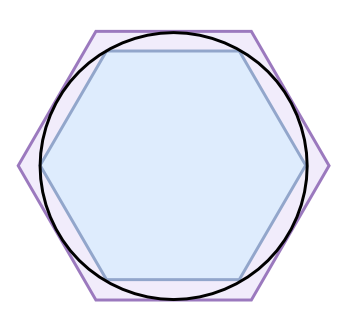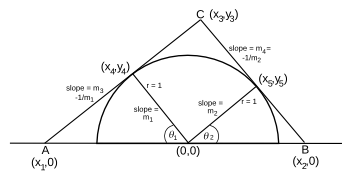### Circumscribing Semicircles with Triangles

August 15, 2016

Humans are fascinated by circles, so much so that many objects are circular in shape, many expressions contain the word, circle, and many English words begin with the prefix, "circum-," derived from the Latin word for circle. One massive online word list has more than 250 words starting with circum, including circumlocution, circumspect, circumstantial, and circumvent.Both sides of a replica of the 3,500 year old Phaistos Disk, a fired clay disk about six inches in diameter. The disk is covered on each side with stamped symbols of an unknown language similar to Linear A and Linear B. (Photo by Maksim, via Wikimedia Commons.)

Geometerss are well acquainted with circles, and also polygons inscribed within, and circumscribed around, circles. At about 250 BC, the Greek mathematician, Archimedes, circumscribed a hexagon and polygons up to 96 sides around a circle to get good estimates of the mathematical constant, pi.

A side of a hexagon circumscribed around a circle of unit diameter is 1/√3, so that its perimeter is 6/√3, or 3.46. A hexagon inscribed in a circle has a perimeter of 3.0 (see figure). The value of pi, Archimedes reasoned, was in between these values. The value found for a circumscribed 96-sided polygon is 22/7, the elementary school approximation of pi (3.1429).Hexagons inscribed within, and circumscribed around, a circle.

The circumscribed hexagon gives an approximate value for pi of 3.46, while the inscribed hexagon gives 3.0. The actual value of pi (3.1415926535...) is between these estimates.

(Via Wikimedia Commons.)

I find plane geometry problems enjoyable, since they are quite simply stated. Some of them are also amenable to a computer solution, so they allow me to practice my (limited) computer programming skill.

In a recent arXiv posting, Jun Li of the Jiangxi University of Science and Technology (Ganzhou, China) presented the problem of finding the triangle of smallest area that can be circumscribed around a semicircle. He did an analytical solution of the problem under a particular constraint, but his paper got me interested in the general problem.

As can be seen in the figure, triangles circumscribed around a semicircle come in many sizes. You can circumscribe right triangles, fat triangles, and tall triangles. The constraint in this case is that the base of the triangle aligns with the base of the semicircle, and the two other sides are tangent to the curve of the semicircle.A variety of triangles circumscribed about a semicircle. (Illustration by the author using Inkscape.)

In searching for the circumscribed triangle of minimum area, I found that it was easiest to specify the angles of the altitudes from the base of the unit semicircle, as shown in the figure. It was then time to extract the long dormant information I had learned in my high school geometry class. As an indication of how long ago that was, I attended my 50th high school reunion last year.(Illustration by the author using Inkscape. Click for larger image.)

Some important ideas of this computation are that the slopes of perpendicular lines are their negative reciprocals, and intersecting points (x,y) are found by equating the line equations, y = mx + b, where m is the slope and b is the y-intercept. The sine and cosine functions are useful for finding the length of the sides of right triangles when an angle and the length of another side are known.

In the past, a computer program of this sort would use a minimum value search algorithm akin to the method of steepest descent. Today's computers are so fast that a lazy programmer can do an exhaustive search in a problem like this, perhaps with a reduction in the variable increments as they get close to the goal; or, the Monte Carlo method that I used, in which you try random values and keep track of how well you're doing. My C language source code, in my usual amateur coding style, can be found here

So what's the result? A proper mathematician might have told me that it was obvious that an isosceles right triangle (θ1 = θ2 = 45°) gives the minimum area. For a semicircle radius of one, the area is 2.0. This can be seen from the contour plot, shown below, that also indicates how slowly the area changes with angle.Contour plot of triangle areas.

(Created from data from the author's program using Gnumeric.)### Reference:

Linked Keywords: Human; circle; English language; prefix; Latin; Internet; online; circumlocution; circumspect; circumstantial; circumvent; replica; Phaistos Disk; furnace; fire; clay; inch; diameter; stamping; stamped; symbol; Linear A; Linear B; Wikimedia Commons; Euclidean geometry; geometer; polygon; inscribed figure; tangential polygon; circumscribe; Anno Domini; BC; Ancient Greece; Greek; mathematician; Archimedes; hexagon; polygon approximation; estimate; mathematical constant; pi; diameter; perimeter; elementary school; approximation; plane geometry; computer; computer programming; arXiv; Jiangxi University of Science and Technology (Ganzhou, China); triangle; area; semicircle; mathematical analysis; constraint; academic publishing; paper; right triangle; base; tangent; curve; Inkscape; angle; height; altitude; information; high school; class reunion; slope; perpendicular; line segment; negative number; multiplicative inverse; reciprocal; intersection; intersecting; point; equating; equation; y-intercept; sine; cosine; trigonometric function; minimum value; algorithm; method of steepest descent; sloth; lazy; programmer; brute-force search; exhaustive search; independent variable; increment; Monte Carlo method; randomness; random value; C programming language; source code; amateur; circumscribe.c; mathematician; isosceles triangle; contour plot; Gnumeric.